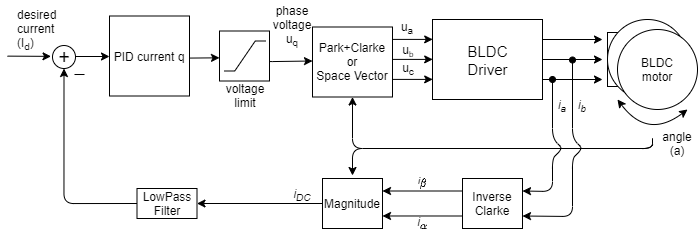# Torque control using DC current

This control loop allows you to run the BLDC motor as it is a current controlled DC motor. This torque control algorithm requires current sensing hardware. The user sets the target current Id to the FOC algorithm calculates the necessary phase voltages ua ,ub and uc in order to maintain it. This mode is enabled by:

// DC current torque control mode
motor.torque_controller = TorqueControlType::dc_current;


## How does it work exactlyThe DC current torque control algorithm reads the phase currents of the BLDC motor (usually ia and ib). Furthermore the algorithm reads the rotor angle a from the position sensor. The phase currents are transformed into the DC current iDC using the Inverse Clarke and Park(simplified) transform. Using the target curren value Id and the measured iDC the PID controller calculates the appropriate voltage Uq to be set to the motor, Ud is kept in 0. Finally FOC algorithm sets the appropriate ua, ub and uc voltages to the motor. FOC algorithm ensures that these voltages generate the magnetic force in the motor rotor exactly with 90 degree offset from its permanent magnetic field, which guarantees maximal torque, this is called commutation.

The assumption of this torque control mode is that the torque generated in the motor is proportional the DC current iDC drawn by the motor (iDC = iq). Therefore by controlling this current we user can control the torque value. This assumption is only true for the low velocities, for higher velocities the id component of the current becomes higher and iDC=iq no longer holds.

## Configuration parameters

In order to make this loop run smoothly the user needs to configure the PID controller parameters of teh PID_current_q and Low pass filter LPF_current_q time constant.

// PID parameters - default
motor.PID_current_q.P = 5;                       // 3    - Arduino UNO/MEGA
motor.PID_current_q.I = 1000;                    // 300  - Arduino UNO/MEGA
motor.PID_current_q.D = 0;
motor.PID_current_q.limit = motor.voltage_limit;
motor.PID_current_q.ramp = 1e6;                  // 1000 - Arduino UNO/MEGA
// Low pass filtering - default
LPF_current_q.Tf= 0.005;                         // 0.01 - Arduino UNO/MEGA


## Torque control example code

A simple example of the DC current based torque control using Inline current sensor and setting the target value by serial command interface.

#include <SimpleFOC.h>

// BLDC motor & driver instance
BLDCMotor motor = BLDCMotor(11);
BLDCDriver3PWM driver = BLDCDriver3PWM(9, 5, 6, 8);

// encoder instance
Encoder encoder = Encoder(2, 3, 500);
// channel A and B callbacks
void doA(){encoder.handleA();}
void doB(){encoder.handleB();}

// current sensor
InlineCurrentSense current_sense = InlineCurrentSense(0.01, 50.0, A0, A2);

// instantiate the commander
Commander command = Commander(Serial);
void doTarget(char* cmd) { command.scalar(&motor.target, cmd); }

void setup() {

// initialize encoder sensor hardware
encoder.init();
encoder.enableInterrupts(doA, doB);
// link the motor to the sensor

// driver config
// power supply voltage [V]
driver.voltage_power_supply = 12;
driver.init();

// current sense init hardware
current_sense.init();
// link the current sense to the motor

// set torque mode:
motor.torque_controller = TorqueControlType::dc_current;
// set motion control loop to be used
motor.controller = MotionControlType::torque;

// foc current control parameters (Arduino UNO/Mega)
motor.PID_current_q.P = 5;
motor.PID_current_q.I= 300;
motor.LPF_current_q.Tf = 0.01;

// use monitoring with serial
Serial.begin(115200);
// comment out if not needed
motor.useMonitoring(Serial);

// initialize motor
motor.init();
// align sensor and start FOC
motor.initFOC();

Serial.println(F("Set the target current using serial terminal:"));
_delay(1000);
}

void loop() {

// main FOC algorithm function
motor.loopFOC();

// Motion control function
motor.move();

// user communication
command.run();
}